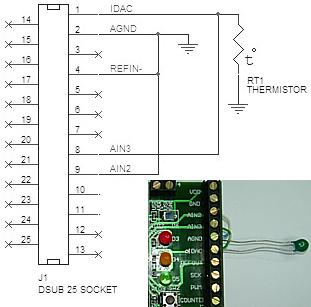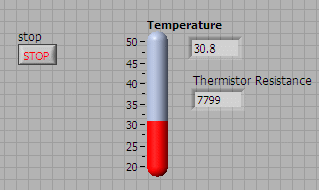# Measure Temperature using Thermistor

Thermistors are widely used because of their sensitivity, small size, ruggedness and low cost. Thermistors have an electrical resistance that varies non-linearly with temperature. The R-T characteristics of most thermistors can be described by the Steinhart-Hart equation:

1/T = A + B*(Ln R) + C*(Ln R)3

T is the absolute temperature (in Kelvin) and A, B, and C are constants which can be determined by measuring three sets of resistance and temperature values during calibration.

Most thermistors have a negative temperature coefficient (NTC), their resistance decreases with increasing temperature. Thermistors are specified according to its nominal resistance at 25 oC and commonly available thermistors range from 250 ohms to 100 kohms

The thermistor that we are using has the following characteristics

• Nominal resistance @ 25 oC: 10 kohms

• negative temperature coefficient (NTC)

• Steinhart-Hart equation parameters:

• A = 0.001129148
• B = 0.000234125
• C = 8.76741E-8As the DAQ module Analog Input measures only voltage, we will need to provide a current source to convert the resistance to voltage. The EMANT300 has an 8 bit current DAC (digital to analog converter). As the DAC has 8 bits resolution, we can drive the resistance from 0 to 1mA in 255 steps with increments of about 39uA.

To measure temperature using the thermistor with the EMANT300 DAQ Training Kit,

• Connect the thermistor to the Light Application Adaptor screw terminals labeled IDAC and AGND
• Connect a wire from IDAC to AIN3
• Connect a wire from AGND to AIN2

The LabVIEW VI that reads the voltage across the thermistor, converts the voltage to resistance and then temperature is called EMANT300 Example Thermistor.VI

## Front Panel## DiagramIn the formula node, the first line calculates the Thermistor resistance. The next line converts from resistance to temperature in Kelvin and finally converts the temperature from Kelvin to Centigrade.